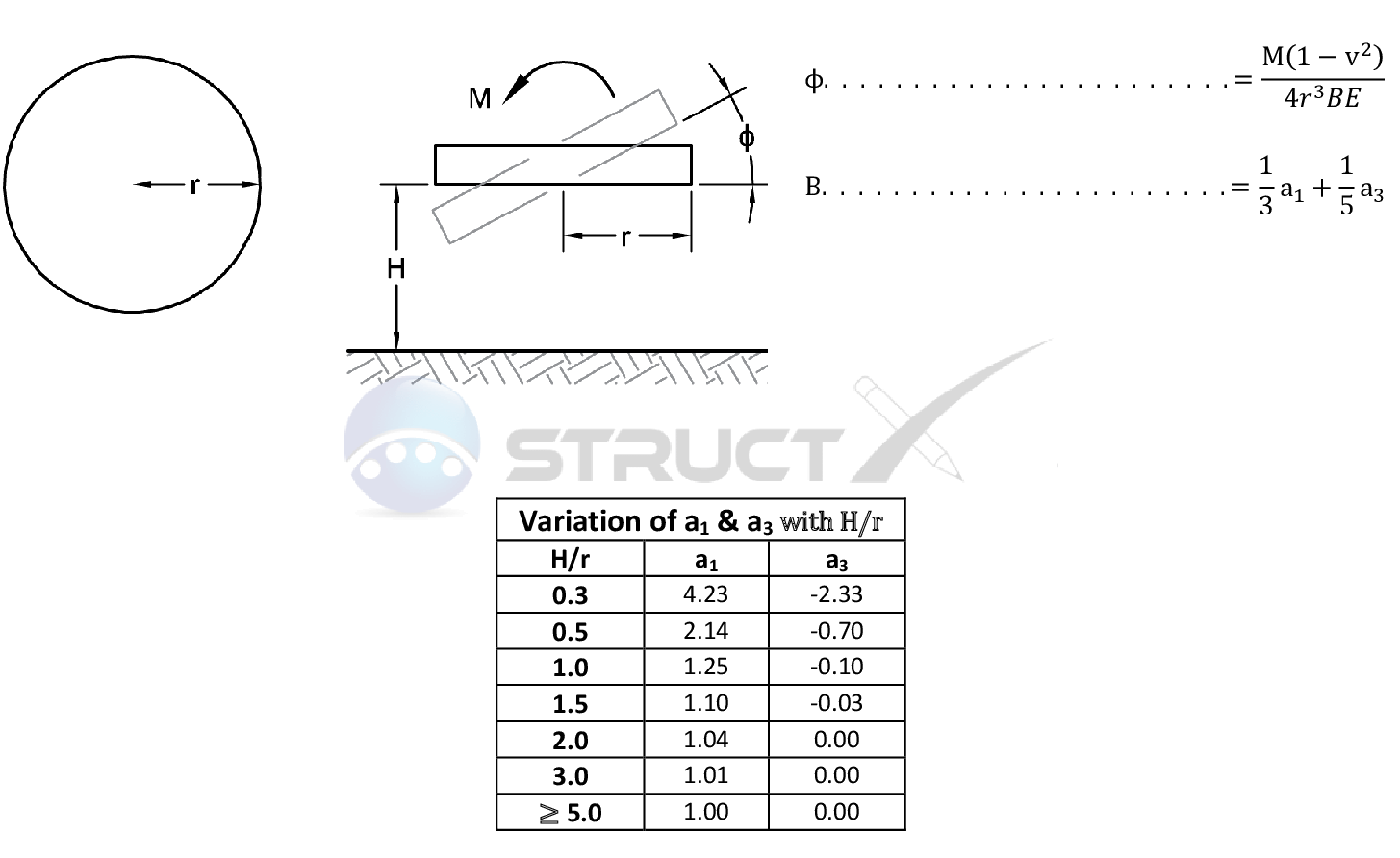# Rotation of a Rigid Circular Footing - Infinite Soil LayerMore Cases# Notation and Units

## Metric and Imperial Units

The above formulas may be used with both imperial and metric units. As with all calculations care must be taken to keep consistent units throughout with examples of units which should be adopted listed below:

# Notation

• B = width of foundation, ft or m
• E = soil modulus, kip/ft2 or kPa
• IB = Boussinesq stress co-efficient
• L = length of loaded area, ft or m
• M = maximum bending moment, kip.ft or kNm
• P = total concentrated load, kip or kN
• w = load per unit length, kip/ft or kN/m
• q = load per unit area, kip/ft2 or kN/m2
• r = horizontal distance to point of interest from load, ft or m